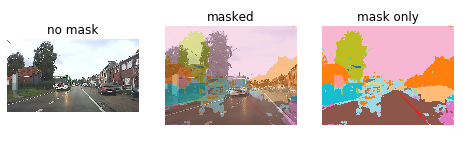# Error when resizing predicted mask

Hi all,

I have trained a model for the camvid dataset (as part of lesson 3) which I exported to my Google Drive.
Then I loaded it with `learn.load` and executed the following:
`pred_mask, lbl, probs = learn.predict(img)`.

The size of `pred_mask` is (720, 960), but my `img` has size (720, 1280).

Now when I try to overlay my image with the predicted mask, it does not fit.
I tried to achieve the overlay with the code from the fast ai docs:
`_,axs = plt.subplots(1,3, figsize=(8,4))`
`img.show(ax=axs, title='no mask')`
`img.show(ax=axs, y=pred_mask, title='masked')`
`pred_mask.show(ax=axs, title='mask only', alpha=1.)`

The results that I get is this:But now I want to resize the mask, so I tried to do this:
`pred_mask.resize((1.0,img.size,img.size))`

After doing so, I got this error: RuntimeError: grid_sampler(): expected input and grid to have same dtype, but input has long and grid has float

So I thought maybe I am doing this wrong and I should apply a transforms, so I did:
`tfms = get_transforms()`
`pred_mask.apply_tfms(tfms,size=(1, img.size, img.size))`

This gives the same error…

So any thoughts on what I am doing wrong, or how to solve this?

Thanks,
Vincent

For the ones looking for an answer to this after me:
I haven’t found the solution yet to the problem described in my post here.
But someone gave me a tip that I shouldn’t resize the mask itself, but rather the input `img`.

So I resized the `img`, next I passed the resized image to the `predict` method and that gave me a good mask which I could overlay as described in the docs.

I am wondering though, are we even able to resize the mask?

2 Likes

I’m experiencing exactly the same issue with you, and haven’t found the way to resize the mask as well.

In my opinion, I think resizing the mask shall be more flexible, as we want to make the prediction more generalizable to different image sizes.

OK, it seems I found the source of the problem and the solution: by casting the dataType of the ImageSegmentation from long to float!

`pred_mask = ImageSegment(pred_mask.data.type(torch.float32)`
`pred_mask.apply_tfms(tfms,size=(1, img.size, img.size),resize_method=ResizeMethod.SQUISH)`
even though `ImageSegment.data` returns a `long` tensor, it stores a `float` tensor in `px` , so I need to call `ImageSegment` on a `float` tensor instead of a `long` .